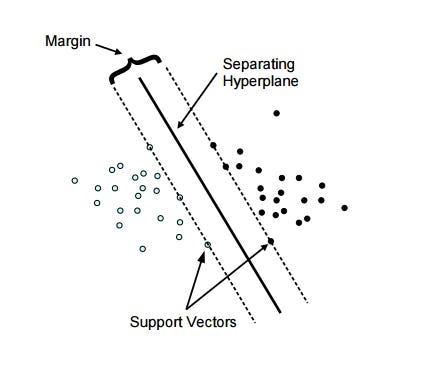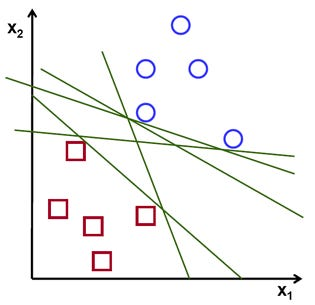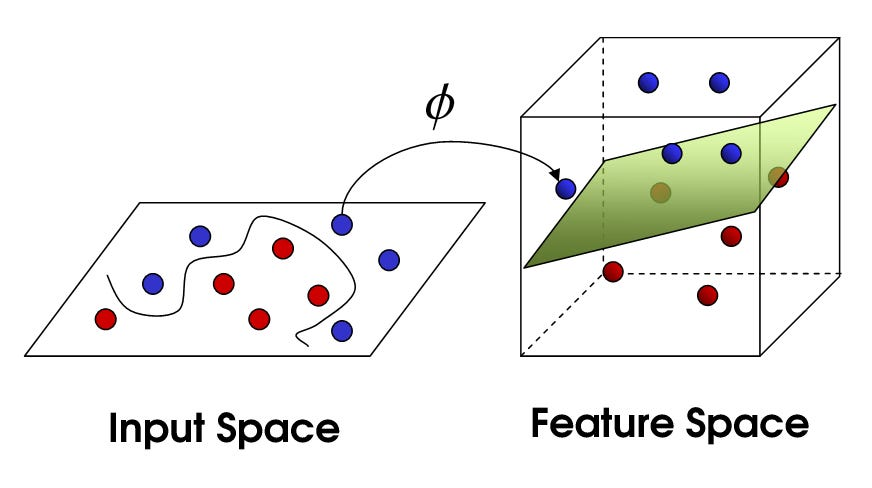# Part I — A high-level overview of Support Vector Machines

Source: Deep Learning on Medium

This post is the first part of a series of posts on Support Vector Machines(SVM) which will give you a general understanding of SVMs and how they work.

### What are SVMs?

SVM is a machine learning technique that can be used for both regression and classification problems. It constructs a hyperplane in a multi-dimensional space to separate a dataset into different classes in the best possible way. Here are some terms you will constantly come across when studying about SVMs,

• Hyperplane — a decision plane that separates and classifies a set of data
• Support vectors — the data points closest to the hyperplane
• Margin — the distance between the hyperplane and the nearest data point from either setFigure 1

### How do they work?

Lets take an example. Say you have two types of data. To separate this data in to two classes a number of different hyperplanes can be used(figure 2). The task of an SVM is to find the optimal plane that best separates the dataset into two classes, that is, the hyperplane for which the margin is maximum.Figure 2

The manner in which an SVM recognizes the optimal hyperplane is as follows,

• Compute the distance between the plane and the support vectors(the margin)
• The optimal hyperplane is the plane which has the maximum distance from the closest data points on either side

### What is the kernel trick?

Sometimes the data given may not be linearly separable. Such problems can’t be solved using a linear hyperplane. In such situations, the SVM uses kernels to transform the input space to a higher dimensional space.Figure 3

A kernel is a function that places a low dimensional plane to a higher dimensional space. This allows the projection of data onto a higher dimensional space where it can be separated using a plane(figure 3). In simple terms, it transforms linearly inseparable data to separable ones by adding more dimension to it.

There are 3 main types of kernels used by SVMs,

• Linear Kernel — The dot product between two given observations
• Polynomial Kernel — Allows curved lines in the input space
• Radial Basis Function(RBF) Kernel — Can create complex regions within the feature space

If you want to know more about the types of kernels and the math behind them I have included some fantastic articles in the reference section. This brings us to the end of this post. Hope this helped you get a high-level understanding of SVMs and how they work.

Until next time, Adios…

References